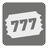Mathematically Lucky Tickets
Simple
English

Sofia cracks open the treasure chest. Inside are slips of paper with six digit numbers on each slip.

“What’s this?” She asks, quizzed. “Nikola, are these what I think they are?”

“They look like lucky tickets! Look at how many there are, there must be hundreds! Any of them could be a winner!”

“Oh man! We could turn them into the lucky ticket commission and collect the reward! I could pay my dad back for the spaceship!”

“Well, not as such. I borrowed the money from my dad on the condition that I’d pay him back. Now maybe I can!”

Stephen sighed. “Well, let’s check the tickets to see if we have a winner.”

“I have an idea. I brought my number cruncher 5000 along. I’ll just write a quick function to find out if we won so we don’t have to do it all manually.” Nikola interjected.

“Quickly, quickly!” Sofia said. “I’m so excited!”

The "Mathematically lucky tickets" concept is similar to the idea of the Russian "Lucky tickets". It refers to the old public transport tickets that had 6-digit numbers printed on them.

You are given a ticket number and the combination of its digits can become a mathematical expression by following these rules.

```    1. The digits of the number can be split into groups of numbers.
2. You cannot change the order of groups or digits.
3. Each group is treated as a one integer number. (1 and 2 would become 12, etc.)
4. Operational signs (+, -, * and /) are placed between the groups.
5. Parenthesis are placed around subexpressions to eliminate any ambiguity
in the evaluation order.

```

For example:

```    * 238756 -> (2 * (38 + ((7 + 5) * 6)))
* 000859 -> (0 + (00 + (8 + (5 + 9))))
* 561403 -> (5 * (6 + (1 + (40 / 3))))

```

The ticket is considered mathematically lucky if no combination of its digits evaluates to 100. For example:

```    * 000000 is obviously lucky, no matter which combination you construct it always
evaluates to zero,
* 707409 and 561709 are also lucky because they cannot evaluate to 100
* 595347 is not lucky: (5 + ((9 * 5) + (3 + 47))) = 100
* 593347 is not lucky: (5 + ((9 / (3 / 34)) - 7)) = 100
* 271353 is not lucky: (2 - (7 * (((1 / 3) - 5) * 3))) = 100

```

The combination has to evaluate to 100 exactly to be counted as unlucky. Fractions can occur in intermediate calculations (like in above examples for 593347 and 271353) but the result must be an integer.

Task: Given a 6-digit number of the ticket, the program should determine whether it is mathematically lucky or not.

Input: 6 digits as a string.

Output: Is it mathematically lucky or not as a boolean.

Example:

```checkio('000000') == True
checkio('707409') == True
checkio('595347') == False
checkio('271353') == False
```

How it is used: This is a nice game to improve mind-calculation skills. If you have coder or math-geek friends, then you can give them this as a challenge. Who’s code will check digits faster than the others? After solving this task you will have the skills to cheat! ;-)

Precondition: |digits| == 6

 Users attempted: 658 Users succeeded: 310 Score for solving: 15 Score for every vote: 7
Player of the MonthPyCharm The Python IDE for Professional Developers. Enjoy productive Python, web and scientific development with PyCharm. Download it now!